# RD Sharma Solutions for Class 10 Maths Chapter 15 Areas Related To Circles Exercise 15.3

Another special part of a circular region is known as the segment of a circle. In this exercise, students will be tested with problems about the segment of a circle and its area. The RD Sharma Solutions Class 10 can be used by students to learn the correct method of solving problems explained in a simple language. In addition, students can also download the RD Sharma Solutions for Class 10 Maths Chapter 15 Areas Related To Circles Exercise 15.3 PDF provided below.

## RD Sharma Solutions for Class 10 Maths Chapter 15 Areas Related To Circles Exercise 15.3 Download PDF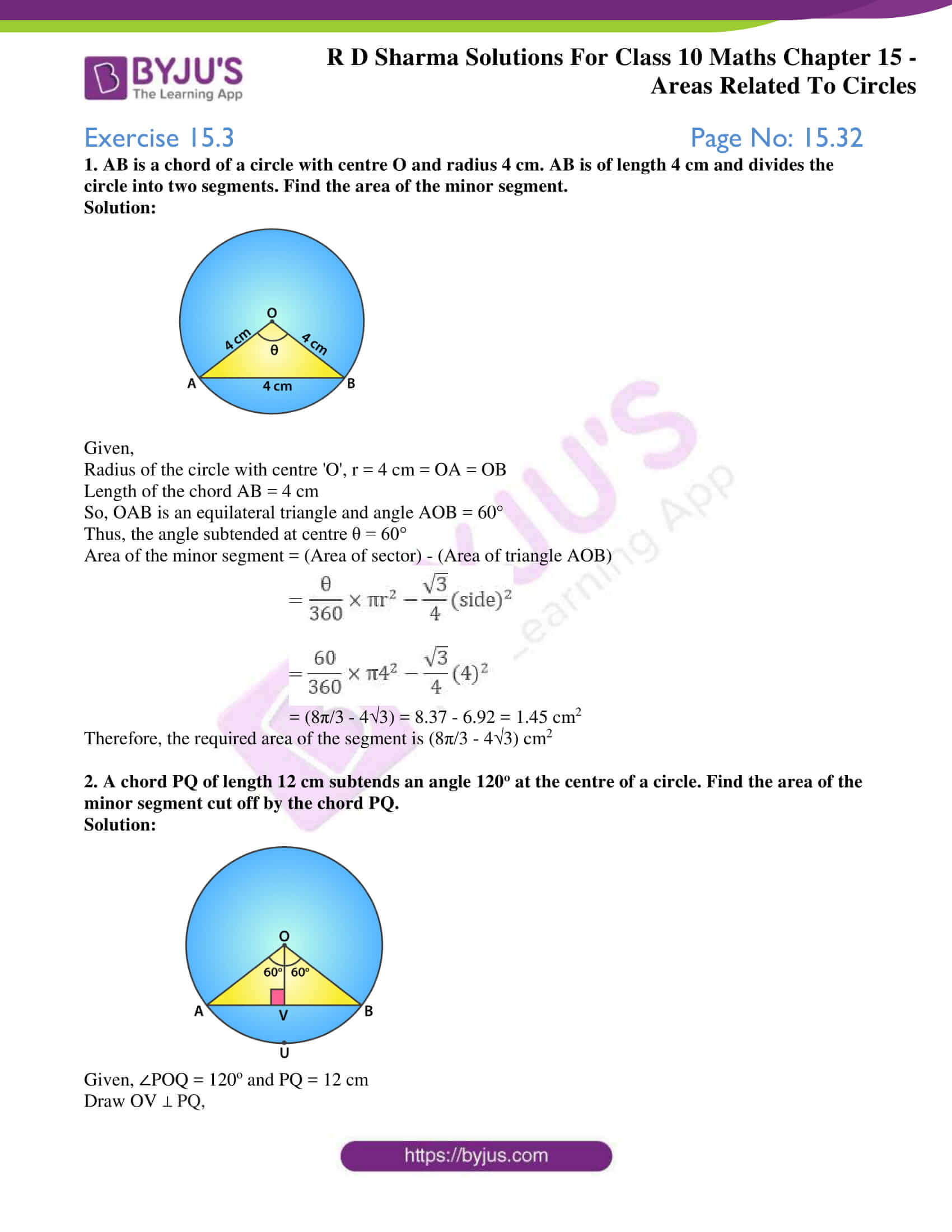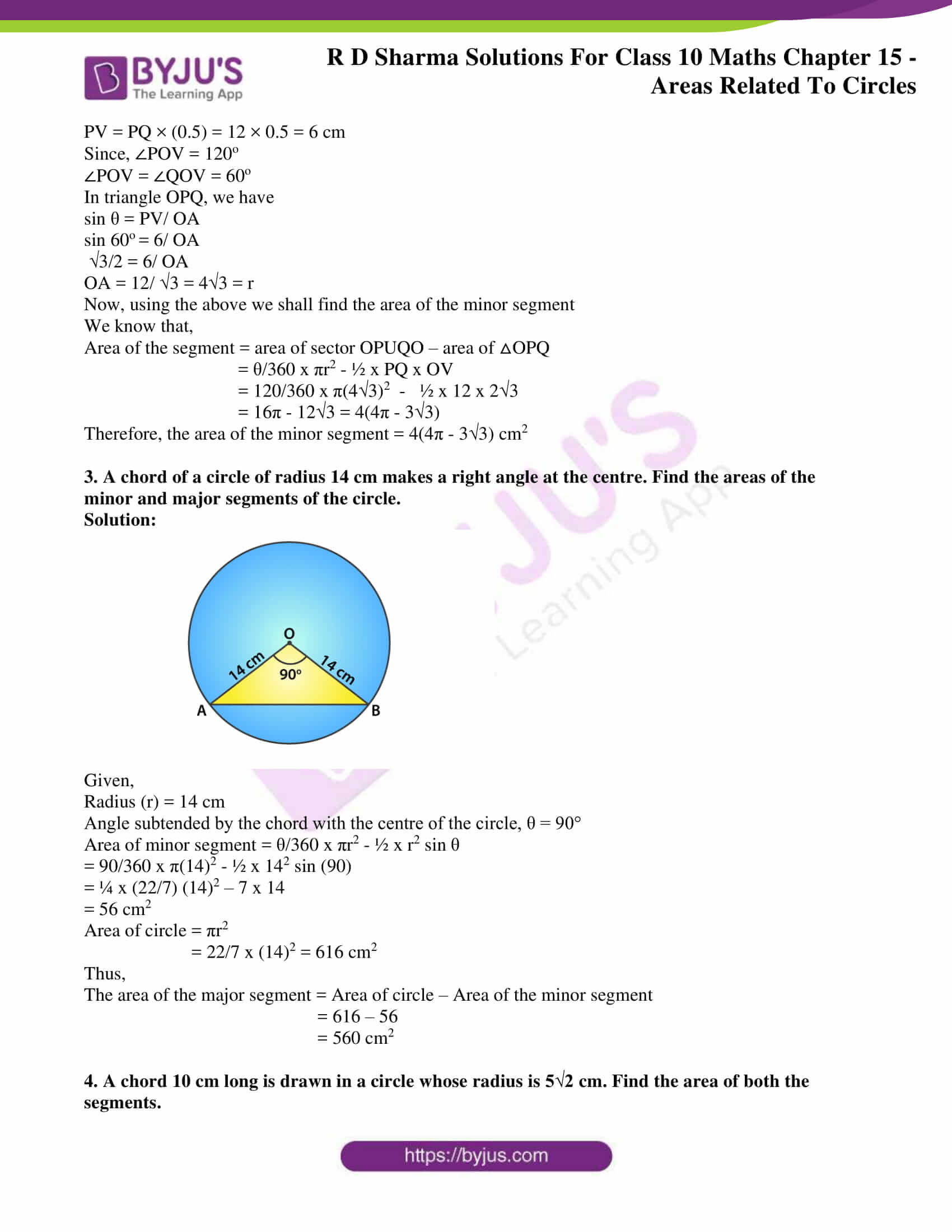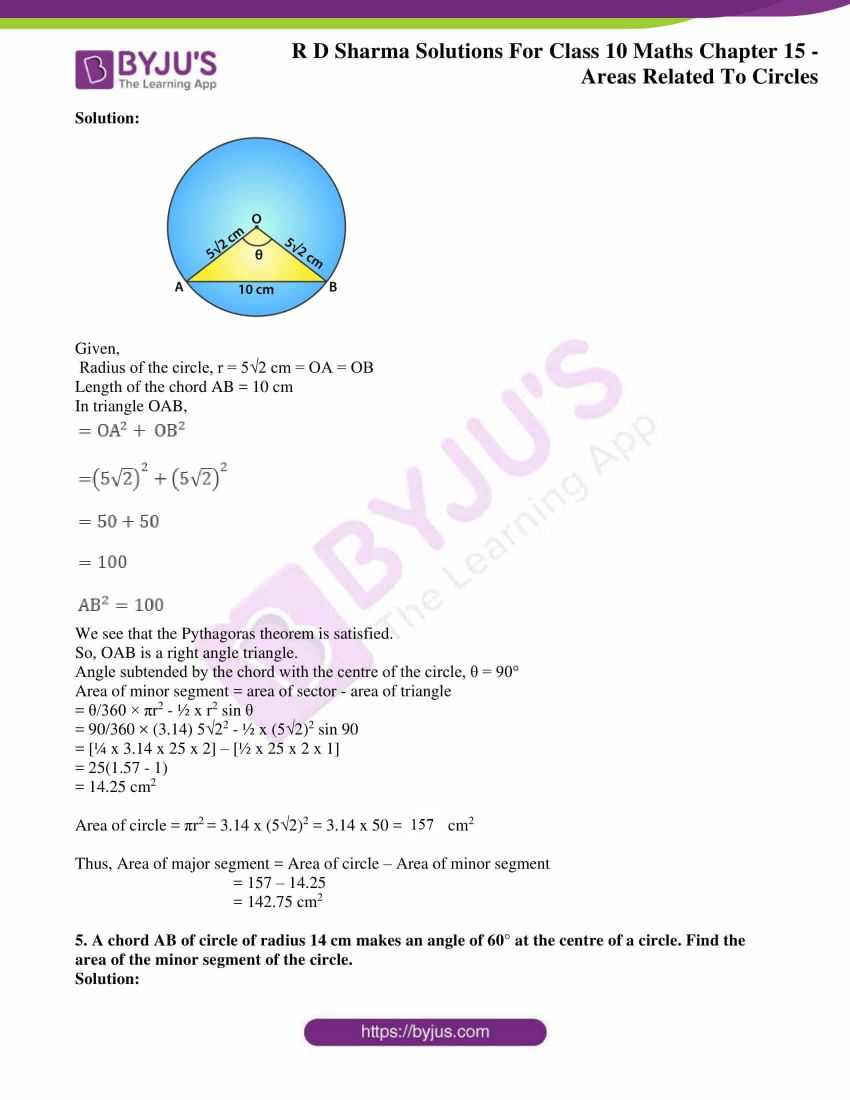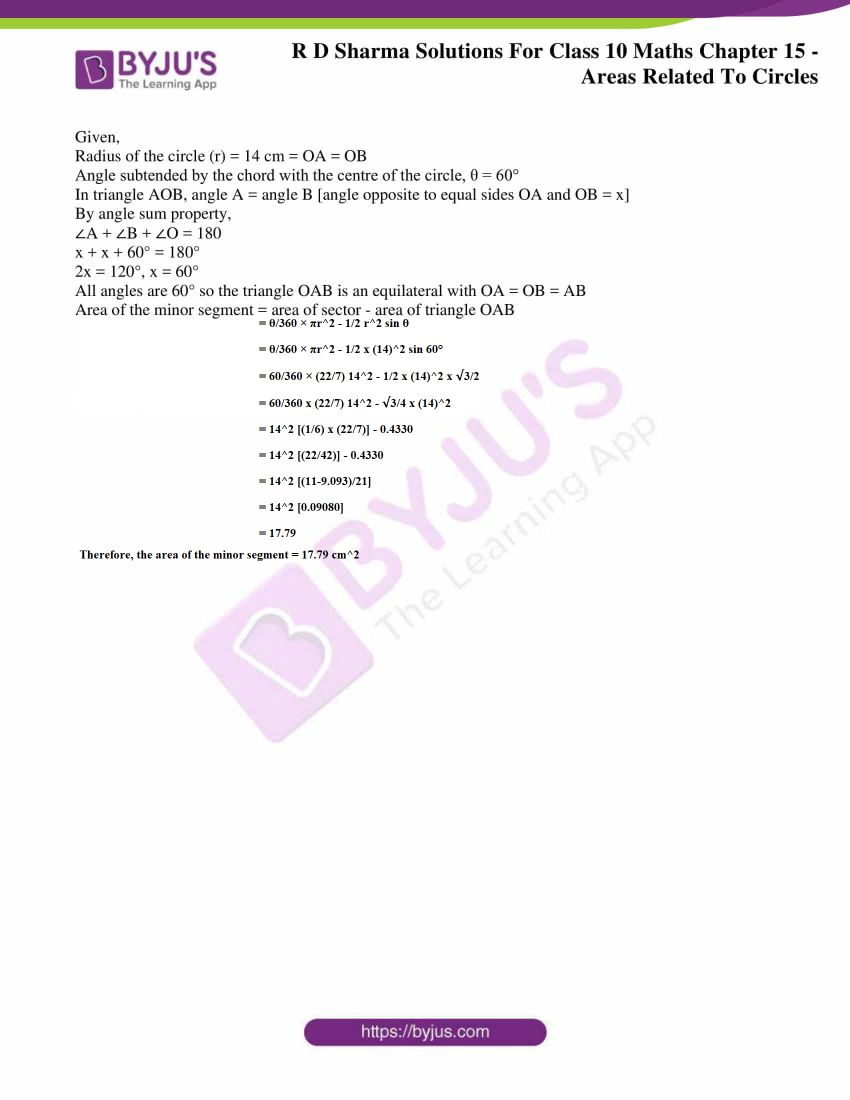### Access RD Sharma Solutions for Class 10 Maths Chapter 15 Areas Related To Circles Exercise 15.3

1. AB is a chord of a circle with centre O and radius 4 cm. AB is of length 4 cm and divides the circle into two segments. Find the area of the minor segment.

Solution: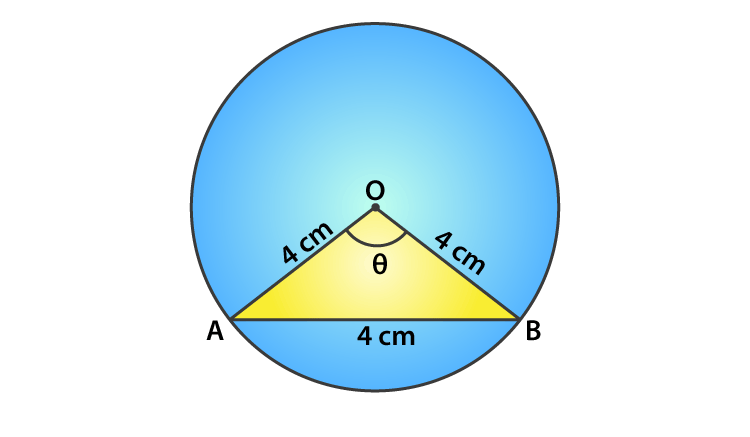Given,

Radius of the circle with centre ‘O’, r = 4 cm = OA = OB

Length of the chord AB = 4 cm

So, OAB is an equilateral triangle and angle AOB = 60°

Thus, the angle subtended at centre θ = 60°

Area of the minor segment = (Area of sector) – (Area of triangle AOB)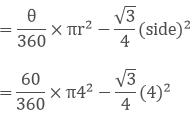= (8π/3 – 4√3) = 8.37 – 6.92 = 1.45 cm2

Therefore, the required area of the segment is (8π/3 – 4√3) cm2

2. A chord PQ of length 12 cm subtends an angle 120o at the centre of a circle. Find the area of the minor segment cut off by the chord PQ.

Solution: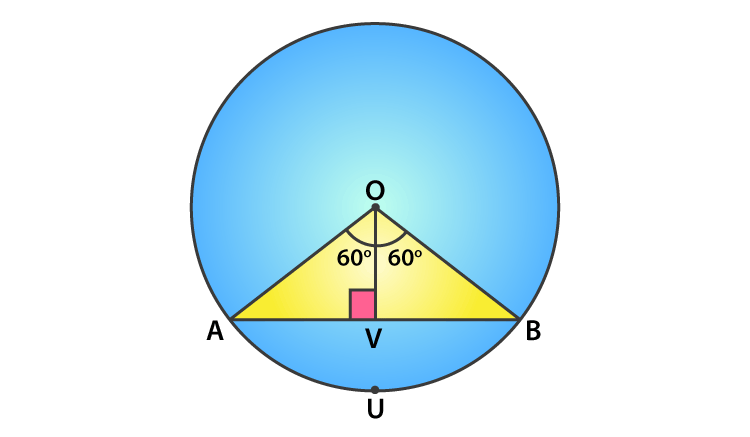Given, ∠POQ = 120o and PQ = 12 cm

Draw OV ⊥ PQ,

PV = PQ × (0.5) = 12 × 0.5 = 6 cm

Since, ∠POV = 120o

∠POV = ∠QOV = 60o

In triangle OPQ, we have

sin θ = PV/ OA

sin 60o = 6/ OA

√3/2 = 6/ OA

OA = 12/ √3 = 4√3 = r

Now, using the above we shall find the area of the minor segment

We know that,

Area of the segment = area of sector OPUQO – area of △OPQ

= θ/360 x πr2 – ½ x PQ x OV

= 120/360 x π(4√3)2 – ½ x 12 x 2√3

= 16π – 12√3 = 4(4π – 3√3)

Therefore, the area of the minor segment = 4(4π – 3√3) cm2

3. A chord of a circle of radius 14 cm makes a right angle at the centre. Find the areas of the minor and major segments of the circle.

Solution: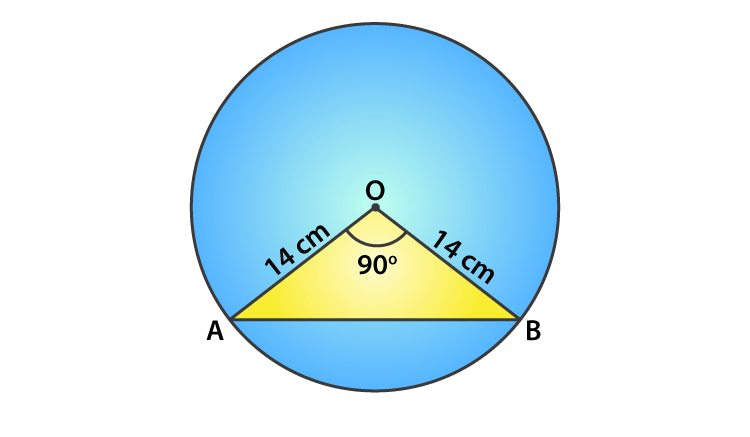Given,

Angle subtended by the chord with the centre of the circle, θ = 90°

Area of minor segment = θ/360 x πr2 – ½ x r2 sin θ

= 90/360 x π(14)2 – ½ x 142 sin (90)

= ¼ x (22/7) (14)2 – 7 x 14

= 56 cm2

Area of circle = πr2

= 22/7 x (14)2 = 616 cm2

Thus,

The area of the major segment = Area of circle – Area of the minor segment

= 616 – 56

= 560 cm2

4. A chord 10 cm long is drawn in a circle whose radius is 5√2 cm. Find the area of both the segments.

Solution: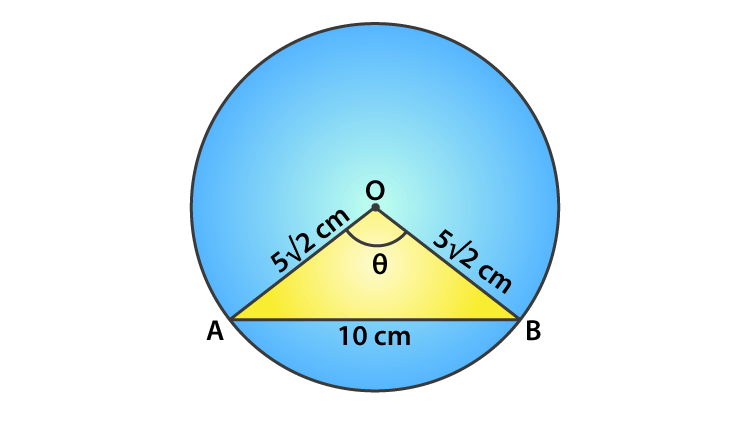Given,

Radius of the circle, r = 5√2 cm = OA = OB

Length of the chord AB = 10 cm

In triangle OAB,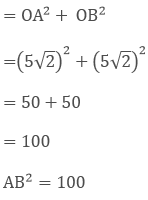We see that the Pythagoras theorem is satisfied.

So, OAB is a right angle triangle.

Angle subtended by the chord with the centre of the circle, θ = 90°

Area of minor segment = area of sector – area of triangle

= θ/360 × πr2 – ½ x r2 sin θ

= 90/360 × (3.14) 5√22 – ½ x (5√2)2 sin 90

= [¼ x 3.14 x 25 x 2] – [½ x 25 x 2 x 1]

= 25(1.57 – 1)

= 14.25 cm2

Area of circle = πr2 = 3.14 x (5√2)2 = 3.14 x 50 = 157 cm2

Thus, Area of major segment = Area of circle – Area of minor segment

= 157 – 14.25

= 142.75 cm2

5. A chord AB of circle of radius 14 cm makes an angle of 60° at the centre of a circle. Find the area of the minor segment of the circle.

Solution:

Given,

Radius of the circle (r) = 14 cm = OA = OB

Angle subtended by the chord with the centre of the circle, θ = 60°

In triangle AOB, angle A = angle B [angle opposite to equal sides OA and OB = x]

By angle sum property,

∠A + ∠B + ∠O = 180

x + x + 60° = 180°

2x = 120°, x = 60°

All angles are 60° so the triangle OAB is an equilateral with OA = OB = AB

Area of the minor segment = area of sector – area of triangle OAB

= θ/360 × πr2 – 1/2 r2 sin θ

= θ/360 × πr2 – 1/2 x (14)2 sin 60°

= 60/360 × (22/7) 142 – 1/2 x (14)2 x √3/2

= 60/360 x (22/7) 142 – √3/4 x (14)2

= 142 [(1/6) x (22/7)] – 0.4330

= 142 [(22/42)] – 0.4330

= 142 [(11-9.093)/21]

= 142 [0.09080]

= 17.79

Therefore, the area of the minor segment = 17.79 cm2